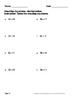One Step Equations - Multiplication & Division WorksheetsSubject
Resource Type
Product Rating
4.0
2 Ratings
File Type

PDF (Acrobat) Document File

Be sure that you have an application to open this file type before downloading and/or purchasing.

11 MB|302 pages
Share
Product Description
One Step Equations - Multiplication and Division Worksheets.
Package includes 150 worksheets.

One-Step Equations - Multiplication (50 Worksheets)
Example:
x * 2 = 6

One-Step Equations - Division (50 Worksheets)
Example:
x ÷ 2 = 4
10 ÷ x = 5

One-Step Equations - Multiplication & Division (50 Worksheets)
Example:
4x = 8
x ÷ 2 = 4
6 ÷ x = 8

You can purchase all One-Step Equations Operations Worksheets (Addition, Subtraction, Multiplication and Division Worksheets) and save your money! One-Step Equations 6\$

----------------------------------

You May Also Like These Worksheets:
One-Step Equations - All Basic Operations
One-Step Equations - Addition & Subtraction
One-Step Equations - Multiplication & Division
Two-Step Equations

You May Also Like These Task Cards:
One Step Equations Task Cards
Two Step Equations Task Cards

Be the first to know about my new discounts, freebies and product launches:
- Look for the green star next to my store logo and click it to become a follower. You will now receive email updates about this store.
- Follow me on Pinterest
Total Pages
302 pages
Included
Teaching Duration
N/A
Report this Resource to TpT
Reported resources will be reviewed by our team. Report this resource to let us know if this resource violates TpT’s content guidelines.